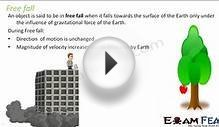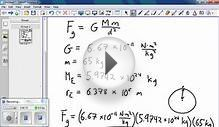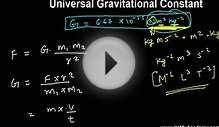# Universal gravitational formula

November 16, 2016States that the gravitational force between two objects is proportional to the product of their masses and inversely proportional to the square of separation between them. This equation is a result of Isaac Newton's Law of Universal Gravitation, which states that quantities of matter attract other matter to it.

The proportionality constant in the equation is called the Universal Gravitational Constant. The value of that constant was determined experimentally by Henry Cavendish in 1798.

This Universal Gravitation Equation originally applied to point masses but was extended to masses of finite size with the assumption that their mass was concentrated at their center of mass.

Questions you may have include:

• What is the Universal Gravitation Equation?
• What is the Universal Gravitational Constant?
• How is the equation an approximation?

### Universal Equation

In 1687, Isaac Newton formulated the, which defines the gravitational force between two objects. The equation is:

F = GMm/R2

where

• F is the force of attraction between two objects in newtons (N)
• G is the Universal Gravitational Constant in N-m2/kg2
• M and M are the masses of the two objects in kilograms (kg)
• R is the separation in meters (m) between the objects, as measured from their centers of mass

This equation has proven highly effective in explaining the forces between objects, as well as leading into the effects of gravity.

### Universal Gravitational Constant

When Newton originally stated the equation, he simply said that F was proportional to Mm/R2. The value of the proportionality constant or Universal Gravitational Constant, G, was not even considered for many years and not officially calculated until 1873, 186 years after Newton defined the equation.

The Cavendish Experiment has since been used to determine Universal Gravitational Constant as:

G = 6.674*10−11 N-m2/kg2

Note: The number 10−11 is 1/1011 or 0.01 with 11 zeros after the decimal point.

(.)

### Check on units

It is important to make sure you are using the correct units for each item in your equation. Check by adding units to the gravitation equation and then seeing that the result is correct:

F N = (G N-m2/kg2)*(M kg)*(M kg)/(R m)2

Just considering the units:

N = (N-m2/kg2)*(kg)*(kg)/(m)2

N = (N)*(m2)*(kg)*(kg)/(m2)*(kg2)

N = N

Thus, the units used are correct.

### Other units

G can also be stated in other terms, depending on its usage. Since a newton (N) equals kg-m/s2, you may also see G defined as:

G = 6.674*10−11 m3/kg-s2

Also, in applications where greater separations are studied, it is more convenient to use kilometers instead of meters. Since 1 m = 10−3 km, the value of G is:

G = 6.674*10−20 km3/kg-s2

When comparing a force in newtons with gravitational force with km, the value is the strange combination of units:

G = 6.674*10−17 N-km2/kg2

You can use whichever set of units that fulfill your requirements.

### Equation an approximation

Newton originally stated the Universal Gravitation Equation as the force between two point masses, separated by R.

### Problem with point masses

A problem exists when considering point masses, and that is the situation when the separation between point masses approaches zero. In such a case, the gravitational force becomes approaches infinity. Since that is impossible, there must be some small separation at which the Universal Gravitation Equation breaks down, perhaps at quantum distances.

Source: www.school-for-champions.com